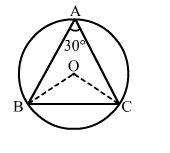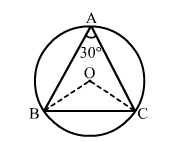# In the given figure, ∠BAC = 30°.

Question:

In the given figure, BAC = 30°. Show that BC is equal to the radius of the circumcircle of ∆ABC whose centre is O.Solution:Join OB and OC.
BOC = 2BAC (As angle subtended by an arc of a circle at the centre is double the angle subtended by the arc at any point on the circumference)

= 2 × 30°       [∵ BAC = 30°]

= 60°           ...(i)

Consider ΔBOC, we have:
OB = OC       [Radii of a circle]
OBC = OCB           ...(ii)
In ΔBOC, we have:
BOC + OBC OCB = 180        (Angle sum property of a triangle)
⇒ 60° + OCB + OCB = 180°       [From (i) and (ii)]
⇒ 2OCB = (180° - 60°) = 120°
⇒ OCB = 60°               ...(ii)
Thus we have:
OBC = OCB = BOC = 60°
Hence, ΔBOC is an equilateral triangle.
i.e., OB = OC = BC
∴ BC is the radius of the circumcircle.